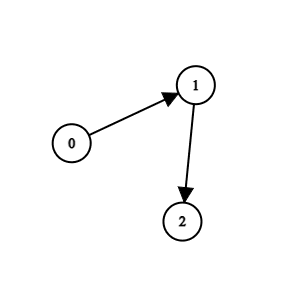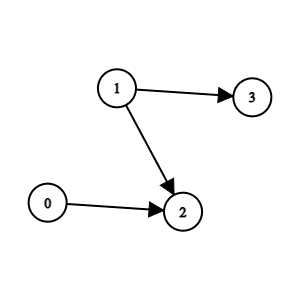# 2924. Find Champion II

## Description

There are n teams numbered from 0 to n - 1 in a tournament; each team is also a node in a DAG.

You are given the integer n and a 0-indexed 2D integer array edges of length m representing the DAG, where edges[i] = [ui, vi] indicates that there is a directed edge from team ui to team vi in the graph.

A directed edge from a to b in the graph means that team a is stronger than team b and team b is weaker than team a.

Team a will be the champion of the tournament if there is no team b that is stronger than team a.

Return the team that will be the champion of the tournament if there is a unique champion, otherwise, return -1.

Notes

• A cycle is a series of nodes a1, a2, ..., an, an+1 such that node a1 is the same node as node an+1, the nodes a1, a2, ..., an are distinct, and there is a directed edge from the node ai to node ai+1 for every i in the range [1, n].
• A DAG is a directed graph that does not have any cycle.

Example 1:Input: n = 3, edges = [[0,1],[1,2]]
Output: 0
Explanation: Team 1 is weaker than team 0. Team 2 is weaker than team 1. So the champion is team 0.


Example 2:Input: n = 4, edges = [[0,2],[1,3],[1,2]]
Output: -1
Explanation: Team 2 is weaker than team 0 and team 1. Team 3 is weaker than team 1. But team 1 and team 0 are not weaker than any other teams. So the answer is -1.


Constraints:

• 1 <= n <= 100
• m == edges.length
• 0 <= m <= n * (n - 1) / 2
• edges[i].length == 2
• 0 <= edge[i][j] <= n - 1
• edges[i] != edges[i]
• The input is generated such that if team a is stronger than team b, team b is not stronger than team a.
• The input is generated such that if team a is stronger than team b and team b is stronger than team c, then team a is stronger than team c.

## Solutions

• class Solution {
public int findChampion(int n, int[][] edges) {
int[] indeg = new int[n];
for (var e : edges) {
++indeg[e];
}
int ans = -1, cnt = 0;
for (int i = 0; i < n; ++i) {
if (indeg[i] == 0) {
++cnt;
ans = i;
}
}
return cnt == 1 ? ans : -1;
}
}

• class Solution {
public:
int findChampion(int n, vector<vector<int>>& edges) {
int indeg[n];
memset(indeg, 0, sizeof(indeg));
for (auto& e : edges) {
++indeg[e];
}
int ans = -1, cnt = 0;
for (int i = 0; i < n; ++i) {
if (indeg[i] == 0) {
++cnt;
ans = i;
}
}
return cnt == 1 ? ans : -1;
}
};

• class Solution:
def findChampion(self, n: int, edges: List[List[int]]) -> int:
indeg =  * n
for _, v in edges:
indeg[v] += 1
return -1 if indeg.count(0) != 1 else indeg.index(0)


• func findChampion(n int, edges [][]int) int {
indeg := make([]int, n)
for _, e := range edges {
indeg[e]++
}
ans, cnt := -1, 0
for i, x := range indeg {
if x == 0 {
cnt++
ans = i
}
}
if cnt == 1 {
return ans
}
return -1
}

• function findChampion(n: number, edges: number[][]): number {
const indeg: number[] = Array(n).fill(0);
for (const [_, v] of edges) {
++indeg[v];
}
let [ans, cnt] = [-1, 0];
for (let i = 0; i < n; ++i) {
if (indeg[i] === 0) {
++cnt;
ans = i;
}
}
return cnt === 1 ? ans : -1;
}